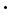Transistor Basics is a free introductory textbook on transistors and their basic applications. See the editorial for more information....# Equivalent Active 'T' Network

Author: Leonard Krugman

While the passive network serves as an interesting introduction to transistor analysis, it does not describe this device completely, because the transistor is known to be an active network. The equivalent circuit of the transistor can be represented in a number of ways; the most widely used configuration is illustrated in Fig. 3-7 (C). The basic difference between the equivalent circuits representing the active network and the passive network is the voltage source e = rmie inserted in the collector arm. In general, the passive network determines three of the characteristics of the active network. In the case under consideration, the input resistance r11, the output resistance r22, and the backward transfer resistance r12 are the same for the passive and active networks shown in Figs. 3-7 (B) and 3-7 (C). The only difference is the value of the forward transfer resistance r21, which in the case of the passive network equals rb, and in the active network equals rm + rb.Fig. 3-7. (A) The basic circuit for transistor four-terminal network analysis.
(B) Transistor equivalent "T" on a passive basis.
(C) Transistor equivalent "T" on an active basis.

, the equations for an active network under ideal conditions (that is, when the resistance of the signal source is zero, and the resistance of the load is infinite) become:

r11 = re + rb

r12 = rb

r21 = rb + rm

r22 = rc + rb

The four parameters in this active network are the emitter resistance re, the base resistance rb, the collector resistance rc, and the voltage source rmie. The parameter rm is represented as a resistance since it acts as the proportionality constant between the input emitter current and the resulting voltage source in the collector arm. The mathematical logic of the resistance rm is easily derived as follows: In preceding chapters, the current gain α was defined as the ratio of the resulting change in collector current to a change in emitter current, α = ic/ie. The equivalent voltage introduced into the collector circuit is e = icrc. Since ic = αie, e = αierc. Since α is a dimensionless parameter, it can be related to the collector resistance by a resistance parameter rm. Thus, α = rm/rc. Substituting this latter equality, e = αierc = rm/rcierc = rmie.

There is no phase inversion in the grounded base connection; a positive signal applied to the emitter produces an amplified positive signal of the same phase at the collector.

Last Update: 2011-01-30